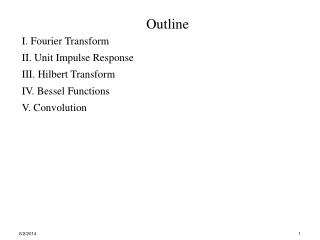Download PresentationOutline I. Fourier Transform II. Unit Impulse Response III. Hilbert Transform IV. Bessel Functions V. ConvolutionOutline I. Fourier Transform II. Unit Impulse Response III. Hilbert Transform IV. Bessel Functions V. Convolution - PowerPoint PPT Presentation

Download PresentationOutline I. Fourier Transform II. Unit Impulse Response III. Hilbert Transform IV. Bessel Functions V. Convolution
An Image/Link below is provided (as is) to download presentation

Download Policy: Content on the Website is provided to you AS IS for your information and personal use and may not be sold / licensed / shared on other websites without getting consent from its author. While downloading, if for some reason you are not able to download a presentation, the publisher may have deleted the file from their server.

- - - - - - - - - - - - - - - - - - - - - - - - - - - E N D - - - - - - - - - - - - - - - - - - - - - - - - - - -
Presentation Transcript

1. Outline I. Fourier Transform II. Unit Impulse Response III. Hilbert Transform IV. Bessel Functions V. Convolution

2. IFT: frequency domain to time domain g(t) = G(f) = • Fourier Transform of all physically realizable time functions will exist • Fourier Transform of all finite energy signals will exist Amplitude Spectrum is |G(f)| plotted vs f Phase Spectrum isθ(f) plotted vs f I. Fourier Transform FT: time domain to frequency domain

3. Duality of Fourier Transform X1(jw) x1(t) 2T1 -T1 T1 1 F[x2(t)] F-1[X2(jw)] F[x1(t)] F-1[X1(jw)] time domain frequency domain x2(t) W/π X2(jw) 1 -W W

4. T0 = 1/f0 t f 50 f0 3f0 f Ts = 1/fs Duality of Fourier Transform between frequency and time domain: (i) time periodic signal yields impulse sequence in frequency domain • (ii) time sampled impulse sequence  yields periodic function in • frequency domain • weighted valued of impulse sequence defines signal completely in • either case

5. w(t) W(f) Rectangular Triangular Sinc Signum Step Impulse Common Functions and their Fourier Transform

6. Constant d ( f ) 1 1 Sinusoid f - f ( j ) ( j ) d - + d + e ( f f ) e ( f f ) c c 2 2 Phasor f ( j ) d - e ( f f ) 0 Guassian - p ( ft ) 2 t e 0 0 1-sided exponential Impulse Train w(t) W(f)

7. g(t) = • II. (t) = delta-dirac function (aka unit impulse) • (t) = 0 except at t = 0 • (t) has unit area under its curve and infinite amplitude • (t) = (-t) – is an even function • sifting or replication property: F-1{δ(f-f0)} = exp(j2πf0t) F{δ(t-t0)} = exp(-j2πft0) F{cos(2πf0t)} = ½ [δ(f - f0) + δ(f + f0)] F{sin(2πf0t)} = -½j [δ(f + f0) - δ(f - f0)]

8. III.Hilbert Transform phase shift x(t) by for positive frequencies phase shift x(t) by for negative frequencies = g(t)sin(2f0t + (t)) t  R no domain change involved (e.g. Fourier Transform) quadrature filter has transfer function H(f) = -jsgn(f) if x(t) = g(t)cos(2f0t + (t)) t  R

9. = Bessel Functions Bessel Differential Equation • equation has • a regular singularity at 0 • an irregular singularity at   Bessel Functions are solutions to Bessel Equation

10. é a a ù ö ö æ æ - = + p r r ÷ ÷ y x C I ç x C Y ç x ê ú 1 q / r 2 q / r è è r r ø ø ë û • In(X) = Bessel Function of the 1st kind • Yn(X) = Bessel Function of the 2nd kind • C1and C2 are constants transformed version of Bessel differential equation (Bowman 1958) solution is

11. Special case ofn = 0 reduces to Bessel Function of the 1st Kind of Integer Order n • Bessel function for real argument x • real-valued and continuously differentiable • bounded in magnitude by 1 • even numbered Bessel Functions are symmetric • odd numbered Bessel Functions are anti-symmetric

12. Plot of Jn(X) =for n = 1,2,3… J0(X) J1(X) J2(X) J3(X) J4(X) X

13. Modified Bessel Function of the 1st Kind of Integer Order n Special case ofn = 0 reduces to • Modified Bessel functionfor real argumentx • real-valued and continuously differentiable • grows exponentially as |x| increases • even numbered Bessel Functions are symmetric • odd numbered Bessel Functions are anti-symmetric

14. Plot of In(X) =for n = 1,2,3… I4(X) I2(X) I0(X) I1(X) I3(X) X

15. IV. Convolution used to determine response to unit impulse • (i) Linear, Time-Invarient system with • impulse response given by h(t) • for arbitrary input x(t) system responseat time t given by y(t) y(t) = or y(t) = = excitation time t= response time t -  = system memory time • current system response = integral over past input signal weighted • by system impulse response System Transfer Function given by H(f) = F[h(t)] = Frequency Response given by Y(f) = H(f)X(f)

16. System Transfer Function given by H(f;) = y(t) = • (ii) LinearTime-Varying Wireless Communications Channel • response depends on the time when unit impulse was applied • impulse response given by h(t;) = system response at time t to unit • impulse applied at time  • - unit impulse applied at t-  • - system response measured at t • for arbitrary input x(t) system responseat time t given by y(t)